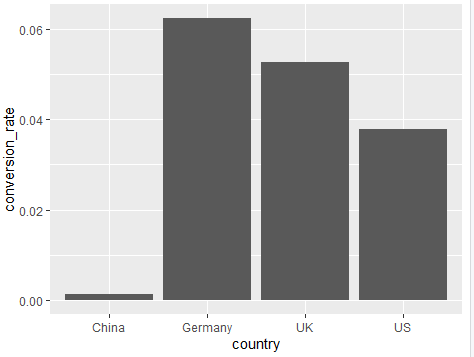3 views

Hi, guys, I am getting this error while plotting a bar graph and I am not able to get rid of it, I have tried both qplot and ggplot but still the same error.

Following is my code

library(dplyr)

library(ggplot2)

#Investigate data further to build a machine learning model

data_country = data %>%

group_by(country) %>%

summarise(conversion_rate = mean(converted))

#Ist method

qplot(country, conversion_rate, data = data_country,geom = "bar", stat ="identity", fill =   country)

#2nd method

ggplot(data_country)+aes(x=country,y = conversion_rate)+geom_bar()

Error:

stat_count() must not be used with a y aesthetic

Data in data_country

country conversion_rate

<fctr>           <dbl>

1   China     0.001331558

2 Germany     0.062428188

3      UK     0.052612025

4      US     0.037800687

The error is coming in the bar chart and not in the dotted chart. Any suggestions would be of great help

by

According to R Documentation,

By default, geom_bar uses stat="count" which makes the height of the bar proportion to the number of cases in each group (or if the weight aethetic is supplied, the sum of the weights). If you want the heights of the bars to represent values in the data, use stat="identity" and map a variable to the y aesthetic.

So if you want to keep the height of the bars according to the conversion rate, use the following code:

library("ggplot2")

data_country <- data.frame(country = c("China", "Germany", "UK", "US"),

conversion_rate = c(0.001331558,0.062428188, 0.052612025, 0.037800687))

ggplot(data_country, aes(x=country,y = conversion_rate)) +geom_bar(stat = "identity")

Output: